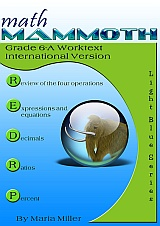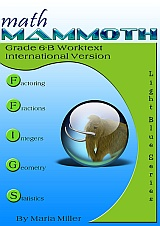Homeschool Buyers Co-op is running a 40% off sale for the large Math Mammoth bundles. The offer expires 8/31/2020.

^
You are here: HomeMM International Version → Grade 6

# Math Mammoth Grade 6 International Version

Math Mammoth Grade 6 International Version is a complete maths program for sixth grade. It consists of two student worktexts (A and B), a separate answer key for each, tests, mixed revisions, and in the download version, an additional worksheet maker (Internet access required) to make extra practice worksheets when needed.179 pages
160 lesson pages178 pages
153 lesson pages

## Prices & ordering

(part A and B worktexts, answer keys,
tests, mixed revisions, worksheet
maker, and Soft-Pak)

Part 6-A only: \$19.75
(part A worktext, part A answer key,
tests, mixed revisions, worksheet
maker, and Soft-Pak)

Part 6-B only: \$19.75
(part B worktext, part B answer key,
tests, mixed revisions, worksheet
maker, and Soft-Pak)

#### Printed copies

You can purchase printed hardcopies at Lulu. The prices below are in USD.

6-A worktext \$16.95
6-B worktext \$16.95
Tests & Mixed Revisions \$8.45

This curriculum is essentially the same as the US version of Math Mammoth Grade 6. The program is customised for the international audience in these aspects:

• The curriculum teaches the metric measurement units. Imperial units, such as inches and pounds, are not used.
• The spelling conforms to British international standards (British English).
• Page (paper) size is A4.
• Large numbers are formatted with a space as the thousands separator (such as 12 394). (The decimals are formatted with a decimal point, like in the US version.)

In money-related word problems, the symbol it uses is "\$" (dollar).

## Introduction

In sixth year, students encounter the beginnings of algebra, algebraic expressions, one-variable equations and inequalities, integers and ratios. We also revise and deepen the students' understanding of rational numbers: both fractions and decimals are studied in depth, while percent is a new topic for 6th year. In geometry, students learn to compute the area of various polygons, and also calculate volume and surface area of various solids. The last major area of study is statistics, where students learn to summarise and describe distributions using both measures of centre and variability.

The year starts out with a revision of the four operations with whole numbers (including long division), place value and rounding. Students are also introduced to exponents and they do some problem solving.

Chapter 2 starts the study of algebra topics, delving first into expressions and equations. Students practise writing expressions in many different ways, and use properties of operations and the idea of maintaining the equality of both sides of an equation to solve simple one-step equations. We also briefly study inequalities and using two variables.

Chapter 3 has to do with decimals. This is a long chapter, as we revise all of decimal arithmetic, just using more decimal digits than in 5th year. Students also convert measuring units in this chapter.

Ratios is a new topic (chapter 4). Students are already familiar with finding fractional parts from earlier years, and now it is time to advance that knowledge into the study of ratios, which arise from dividing a quantity into many equal parts. We study such topics as rates, unit rates, equivalent ratios and problem solving using bar models.

Percent (chapter 5) is an important topic to understand thoroughly, because of its many applications in real life. The goal of this chapter is to develop a basic understanding of percent, to see percentages as decimals and to learn to calculate discounts.

Chapter 6 first revises prime factorisation and then applies those principles to using the greatest common factor to simplify fractions and the least common multiple to find common denominators. After a thorough revision of the fraction operations from fifth year (addition, subtraction and multiplication), Chapter 7, Fractions, then focuses on the new topic for sixth year: the division of fractions. The chapter also includes ample practise in solving problems with fractions.

Chapter 8 introduces students to integers (signed numbers). They plot points in all four quadrants of the coordinate plane, reflect and translate simple figures, and learn to add and subtract with negative numbers. (The multiplication and division of integers will be studied in 7th year.)

The next chapter, Geometry, focuses on calculating the area of polygons. After a brief revision of terminology for triangles and quadrilaterals and drawing fundamentals, this new topic is presented simply in a logical progression: first, the area of a right triangle; next, the area of a parallelogram; then, the area of any triangle; and finally, the area of a polygon. The chapter then expands the concepts learned into developing nets for simple solids to calculate their surface area and to figuring the volume of rectangular prisms with edges of fractional length.

The final chapter is about statistics. Beginning with the concept of a statistical distribution, students learn about measures of centre and measures of variability. They also learn how to make dot plots, histograms, boxplots, and stem-and-leaf plots as ways to summarise and analyse distributions.

Please also see the table of contents for 6-A and 6-B (in the sample), which will let you see the topics covered in more detail.

Some main features of Math Mammoth complete curriculum (Light Blue series) are:

• focuses on understanding of mathematical concepts
• uses clear explanations, lots of visual exercises and pattern exercises
• mastery oriented: concentrates at length on a topic, with very few topics per grade
• emphasizes mental maths and developing number sense
• very little teacher preparation needed

The downloadable version includes a worksheet maker (Internet access required) that lets you make extra practice worksheets for many of the topics in the curriculum.

The PDFs in the download version are enabled for annotation. This means that if you prefer, the student can fill them in on the computer, using the typewriter and drawing tools in Acrobat Reader version 9 or greater.

BONUS! With the download version, you will also get Soft-Pak programs completely FREE. The 6 programs in Soft-Pak (4 math, 1 language arts, 1 list maker) offer both on-screen and printable activities in a low-graphic, high content format. Read more and see screenshots.

## Review

Review: Math Mammoth International Light Blue — a review by Annette V at A Net in Time blog.

## How and where to order

You can buy Math Mammoth International Version:

• As a digital version on this site (Mathmammoth.com) — using the "Add to cart" buttons.
• As a print version at Lulu. Follow the links from this site to Lulu.

Also available:

Math Mammoth Grades 1-3 Bundle, International Version, \$79 USD

Get grades 1-3 in a discounted bundle — 33% off! This means you save \$39.50 USD (or the equivalent in your currency).

Math Mammoth Grades 4-7 Bundle, International Version, \$105 USD

Get grades 4-7 in a discounted bundle — 33% off! This means you save \$53 USD (or the equivalent in your currency).

By purchasing any of the books, permission IS granted for the purchaser (teacher or parent) to reproduce this material to be used with his/her students in a teaching situation; not for commercial resale. Please note: you are not permitted to share the material with other teachers or parents.

In other words, you are permitted to make copies for the students/children you are teaching, but not for other teachers' usage.

WAIT!

Receive my monthly collection of math tips & resources directly in your inbox — and get a FREE Math Mammoth book!You can unsubscribe at any time.

### Math Mammoth Tour

Confused about the different options? Take a virtual email tour around Math Mammoth! You'll receive:

An initial email to download your GIFT of over 400 free worksheets and sample pages from my books. Six other "TOURSTOP" emails that explain the important things and commonly asked questions concerning Math Mammoth curriculum. (Find out the differences between all these different-colored series!)

This way, you'll have time to digest the information over one or two weeks, plus an opportunity to ask me personally about the curriculum.
A monthly collection of math teaching tips & Math Mammoth updates (unsubscribe any time)### "Mini" Math Teaching Course

This is a little "virtual" 2-week course, where you will receive emails on important topics on teaching math, including:

- How to help a student who is behind
- Troubles with word problems
- Teaching multiplication tables
- Why fractions are so difficult
- The value of mistakes
- Should you use timed tests
- And more!

A GIFT of over 400 free worksheets and sample pages from my books right in the very beginning.A monthly collection of math teaching tips & Math Mammoth updates (unsubscribe any time)
Enter your email to receive math teaching tips, resources, Math Mammoth news & sales, humor, and more! I tend to send out these tips about once monthly, near the beginning of the month, but occasionally you may hear from me twice per month (and sometimes less often).• A GIFT of over 400 free worksheets and sample pages from my books.